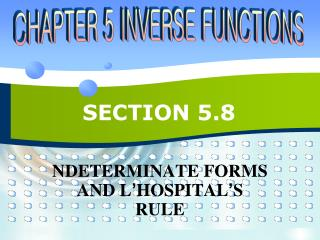DownloadDownload PresentationSECTION 5.8

SECTION 5.8

Download PresentationSECTION 5.8

- - - - - - - - - - - - - - - - - - - - - - - - - - - E N D - - - - - - - - - - - - - - - - - - - - - - - - - - -
Presentation Transcript

1. SECTION 5.8 NDETERMINATE FORMS AND L’HOSPITAL’S RULE

2. L’HOSPITAL’S RULE • Suppose f and g are differentiable and g’(x) ≠ 0 on an open interval I that contains a (except possibly at a). Supposeor that • In other words, we have an indeterminate form of type or ∞/∞. • Then,if the limit on the right exists (or is ∞ or – ∞). 5.8

3. Example 1 • Find • SOLUTION • Thus, we can apply l’Hospital’s Rule: 5.8

4. Example 2 • Calculate • SOLUTION • We have • So, l’Hospital’s Rule gives: 5.8

5. Example 2 SOLUTION • As ex→ ∞ and 2x → ∞ as x → ∞, the limit on the right side is also indeterminate. • However, a second application of l’Hospital’s Rule gives: 5.8

6. Example 3 • Calculate • SOLUTION • As ln x→ ∞ and as x → ∞, l’Hospital’s Rule applies: • Notice that the limit on the right side is now indeterminate of type . 5.8

7. Example 3 SOLUTION • However, instead of applying the rule a second time as we did in Example 2, we simplify the expression and see that a second application is unnecessary: 5.8

8. Example 4 • Find • SOLUTION • Noting that both tan x–x→ 0 and x3 → 0 as x → 0, we use l’Hospital’s Rule: 5.8

9. Example 4 SOLUTION • As the limit on the right side is still indeterminate of type , we apply the rule again: • Since , we simplify the calculation by writing: 5.8

10. Example 4 SOLUTION • We can evaluate this last limit either by using l’Hospital’s Rule a third time or by writing tan x as (sin x)/(cos x) and making use of our knowledge of trigonometric limits. 5.8

11. Example 4 SOLUTION • Putting together all the steps, we get: 5.8

12. Example 5 • Find • SOLUTION • If we blindly attempted to use l-Hospital’s rule, we would get: 5.8

13. Example 5 SOLUTION • This is wrong. • Although the numerator sin x→ 0 as x → p–, notice that the denominator (1 – cos x) does not approach 0. • So, the rule can’t be applied here. • The required limit is, in fact, easy to find because the function is continuous at p and the denominator is nonzero there: 5.8

14. Example 6 • Evaluate • SOLUTION • The given limit is indeterminate because, as x→ 0+, the first factor (x) approaches 0, whereas the second factor (ln x) approaches – ∞. 5.8

15. Example 6 SOLUTION • Writing x = 1/(1/x), we have 1/x→ ∞ as x → 0+. • So, l’Hospital’s Rule gives: 5.8

16. Example 7 • Compute • SOLUTION • First, notice that sec x → ∞ and tan x → ∞ as x → (p/2)–. • So, the limit is indeterminate. 5.8

17. Example 7 SOLUTION • Here, we use a common denominator: • Note that the use of l’Hospital’s Rule is justified because 1 – sin x→ 0 and cos x → 0 as x → (p/2)–. 5.8

18. INDETERMINATE POWERS • Several indeterminate forms arise from the limit 5.8

19. Example 8 • Calculate • SOLUTION • First, notice that, as x→ 0+, we have 1 + sin 4x → 1 and cot x → ∞. • So, the given limit is indeterminate. • Let y = (1 + sin 4x)cot x • Then, ln y = ln[(1 + sin 4x)cot x] = cot x ln(1 + sin 4x) 5.8

20. Example 8 SOLUTION • So, l’Hospital’s Rule gives: 5.8

21. Example 8 SOLUTION • So far, we have computed the limit of ln y. • However,what we want is the limit of y. • To find this, we use the fact that y =eln y: 5.8

22. Example 9 • Find • SOLUTION • Notice that this limit is indeterminate since 0x = 0 for any x > 0 but x0 = 1 for any x ≠ 0. 5.8

23. Example 9 SOLUTION • We could proceed as in Example 8 or by writing the function as an exponential: xx = (eln x)x = ex ln x • In Example 6, we used l’Hospital’s Rule to show that • Therefore, 5.8